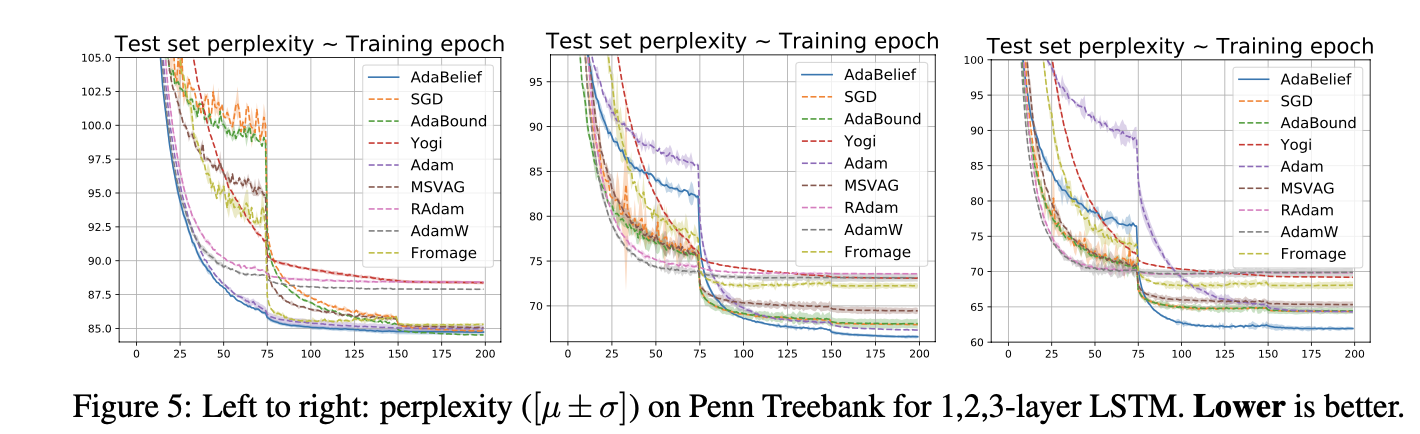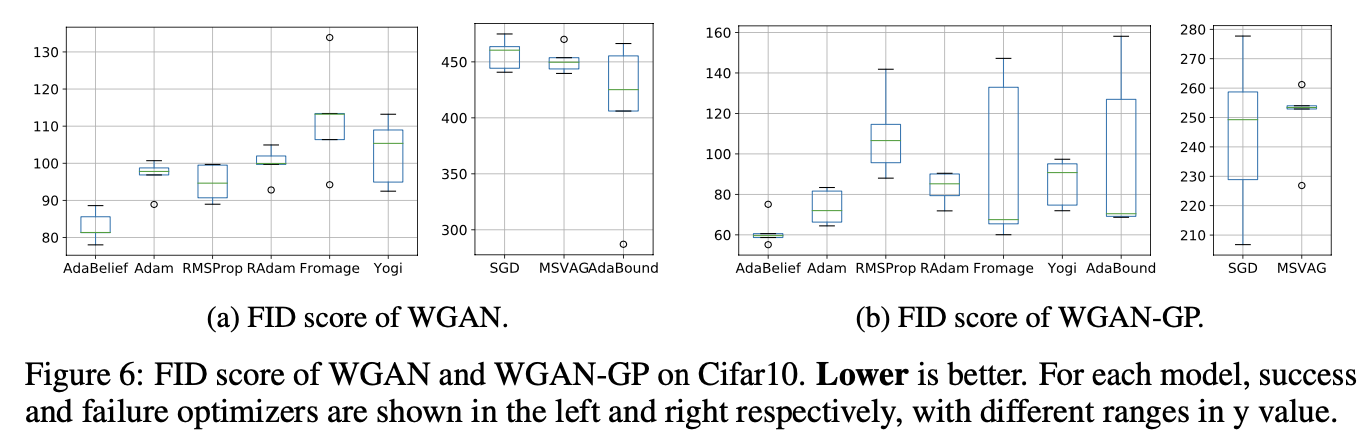## Algorithm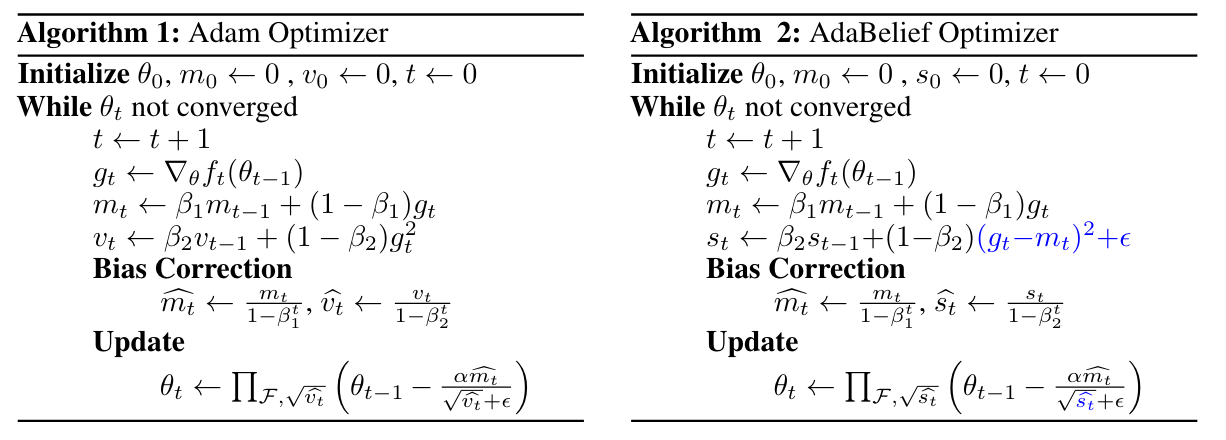Adam and AdaBelief are summarized in Algo.1 and Algo.2, where all operations are element-wise, with differences marked in blue. Note that no extra parameters are introduced in AdaBelief. For simplicity, we omit the bias correction step. Specifically, in Adam, the update direction is $$m_t/\sqrt{v_t}$$, where $$v_t$$ is the EMA (Exponential Moving Average) of $$g_t^2$$; in AdaBelief, the update direction is $$m_t/\sqrt{s_t}$$, where $$s_t$$ is the EMA of $$(g_t - m_t)^2$$. Intuitively, viewing $$m_t$$ as the prediction of $$g_t$$, AdaBelief takes a large step when observation $$g_t$$ is close to prediction m_t, and a small step when the observation greatly deviates from the prediction.

#### AdaBelief considers the curvature of loss function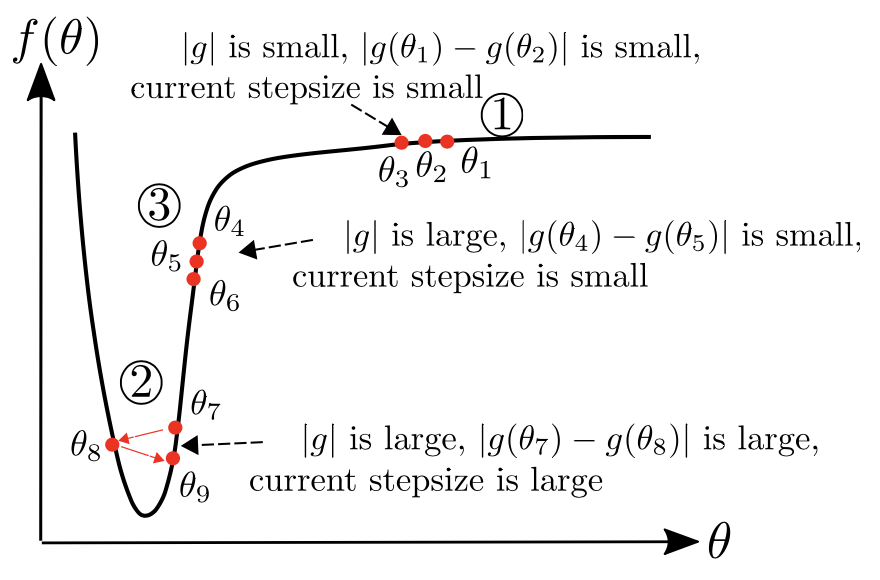An ideal optimizer considers curva- ture of the loss function, instead of taking a large (small) step where the gradient is large (small). In region 3 , we demonstrate AdaBelief’s advantage over Adam in the “large gradient, small curvature” case. In this case, $$\vert g_t \vert$$ and $$v_t$$ are large, but $$\vert g_t − g_{t-1} \vert$$ and $$\vert s_t \vert$$ are small; this could happen because of a small learning rate $$\alpha$$. In this case, an ideal optimizer should increase its stepsize. SGD uses a large stepsize (∼ $$\alpha \vert g_t \vert$$); in Adam, the denominator $$\sqrt{v_t}$$ is large, hence the stepsize is small; in AdaBelief, denominator $$\sqrt{s_t}$$ is small, hence the stepsize is large as in an ideal optimizer.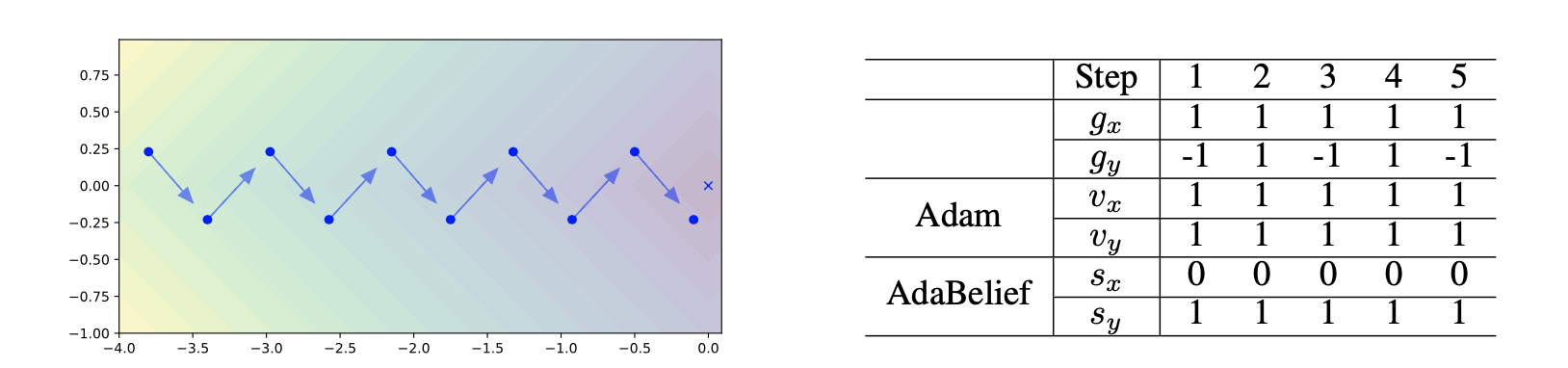Left: Consider $$f(x,y) = \vert x \vert + \vert y \vert$$. Blue vectors represent the gradient, and the cross represents the optimal point. The optimizer oscillates in the y direction, and keeps moving forward in the x direction. Right: Optimization process for the example on the left. Note that denominator $$\sqrt{v_{t,x}} = \sqrt{v_{t,y}}$$ for Adam, hence the same stepsize in x and y direction; while $$\sqrt{s_{t,x}} < \sqrt{v_{t,y}}$$ , hence AdaBelief takes a large step in the x direction, and a small step in the y direction.

#### Update direction in Adam is close to “sign descent” in low-variance case

Under the following assumptions: (1) assume $$g_t$$ is drawn from a stationary distribution, hence after bias correction, $$\mathbb{E} v_t= (\mathbb{E} g_t )^2 + \mathbf{Var} g_t$$. (2) low-noise assumption, assume $$(\mathbb{E} g_t)^2 \gg \mathbf{Var} g_t$$, hence we have $$\mathbb{E}g_t / \sqrt{ \mathbb{E} v_t } \approx \mathbb{E} g_t / \sqrt{(\mathbb{E} g_t)^2} = sign( \mathbb{E} g_t)$$. (3) low-bias assumption, assume $$\beta_1^t$$ ($$\beta_1$$ to the power of $$t$$) is small, hence $$m_t$$ as an estimator of $$\mathbb{E}g_t$$ has a small bias $$\beta_1^t \mathbb{E} g_t$$. Then
$$\begin{equation} \Delta \theta_t^{Adam} = - \alpha \frac{m_t}{\sqrt{v_t} + \epsilon} \approx - \alpha \frac{\mathbb{E} g_t}{ \sqrt{(\mathbb{E}g_t)^2 + \mathbf{Var} g_t } + \epsilon} \approx - \alpha \frac{\mathbb{E} g_t}{ \vert \vert \mathbb{E}g_t \vert \vert } = - \alpha \ \mathrm{sign}( \mathbb{E} g_t ) \end{equation}$$
In this case, Adam behaves like a sign descent''; in 2D cases the update is $$\pm 45^{\circ}$$ to the axis, hence deviates from the true gradient direction. The sign update'' effect might cause the generalization gap between adaptive methods and SGD (e.g. on ImageNet). For AdaBelief, when the variance of $$g_t$$ is the same for all coordinates, the update direction matches the gradient direction; when the variance is not uniform, AdaBelief takes a small (large) step when the variance is large (small).

## Experiments

#### Image Classification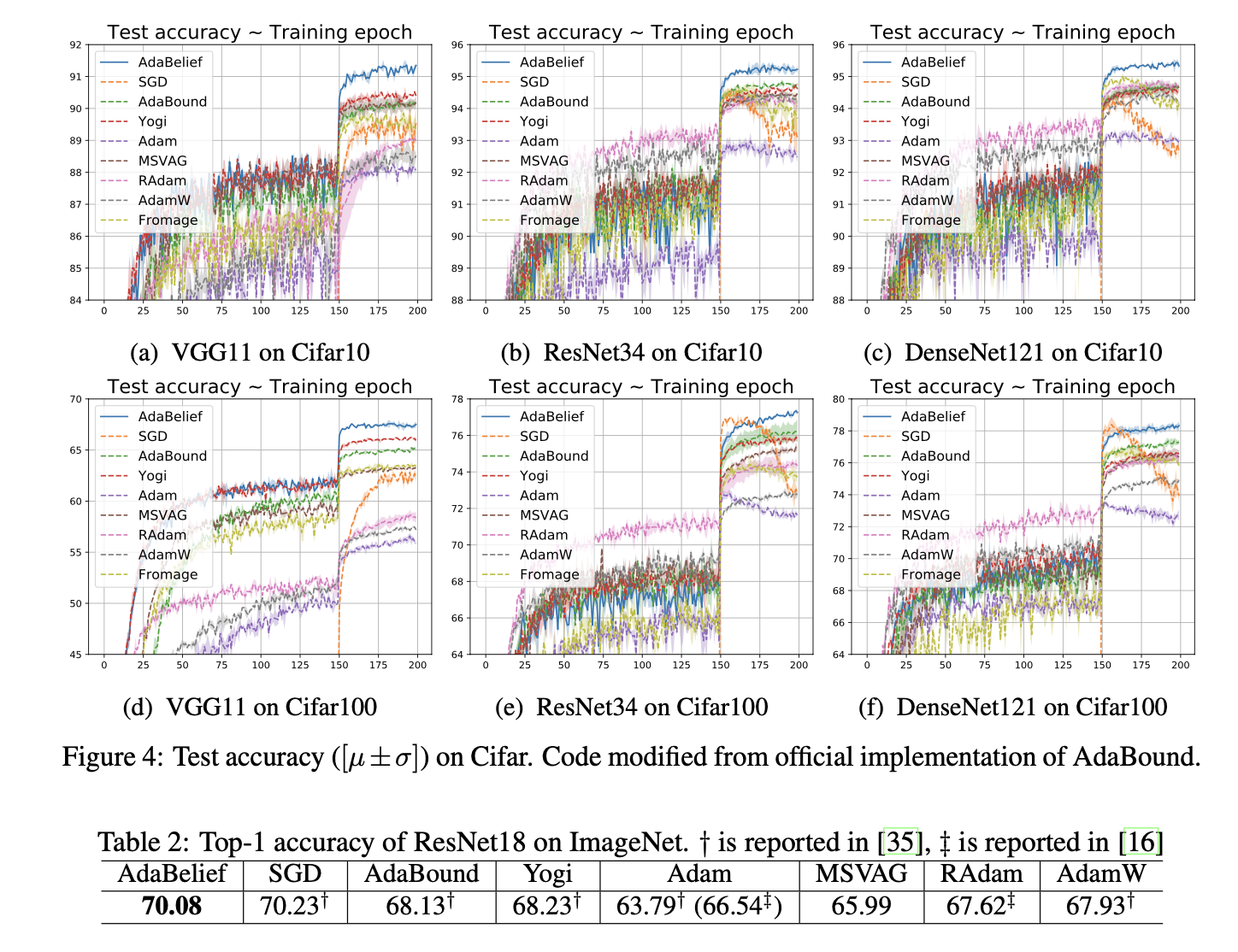#### Time-series Modeling